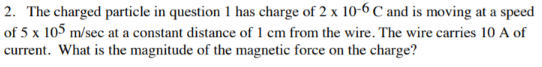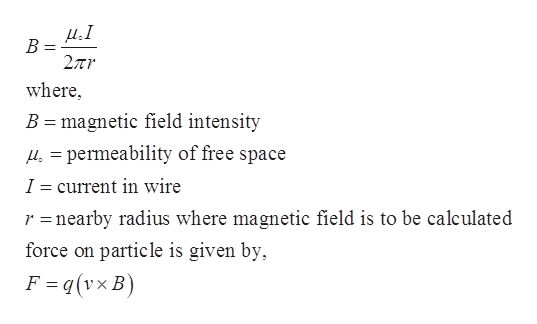# 2. The charged particle in question 1 has charge of 2 x 10-6 C and is moving at a speedof 5 x 105 m/sec at a constant distance of 1 cm from the wire. The wire carries 10 A ofcurrent. What is the magnitude of the magnetic force on the charge?

Question
1 viewshelp_outlineImage Transcriptionclose2. The charged particle in question 1 has charge of 2 x 10-6 C and is moving at a speed of 5 x 105 m/sec at a constant distance of 1 cm from the wire. The wire carries 10 A of current. What is the magnitude of the magnetic force on the charge? fullscreen
check_circle

Step 1

Given:

Charge on particle (q) = 2×10-6 C

Velocity of particle (v) = 5×105 m/s

Current in wire (i) = 10 A

Charge moving at 1cm (say r) from wire.

Step 2

Formula used:

Magnitude of magnetic field generated by current carrying wire is given by,help_outlineImage TranscriptioncloseД.I В 2πη where B magnetic field intensity pemeability of free space I current in wire r nearby radius where magnetic field is to be calculated force on partic le is given by, F =q(vxB) fullscreen
Step 3

Let, charged particle is moving in the direction of flow of current.

The ma...

### Want to see the full answer?

See Solution

#### Want to see this answer and more?

Solutions are written by subject experts who are available 24/7. Questions are typically answered within 1 hour.*

See Solution
*Response times may vary by subject and question.
Tagged in

### Electric Charges and Fields